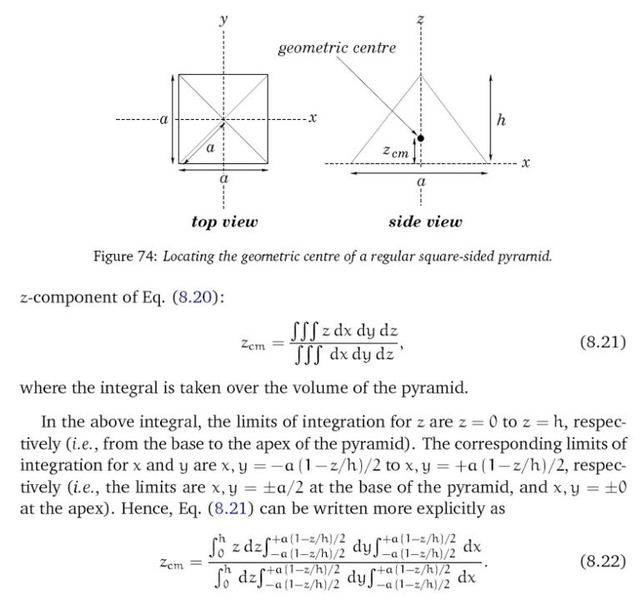# Lower and upper limits for a volume integral

• chrisyuen

## Homework StatementI read an e-book about the classical mechanics and didn't know how to find the lower and upper limits for a volume integral.

## Homework Equations

Perhaps, it may be related to the use of similar triangle.

## The Attempt at a Solution

I calculated the x value only (y is the same as x because of symmetry)

z/h = x/(a/2) = 2x/a

x = az/2h (distance)

Lower Limit = -az/2h
Upper Limit = az/2h

Can anyone tell me how to obtain the same values as the one given by the author?

Thank you very much!

f(z) = (1 - z/h) is a function that goes linearly from 1 down to zero as z goes from 0 to h:

z = 0 --> f(z) = 1
z = h --> z/h = 1 --> f(z) = 0

Multiply by the desired width at the base (a/2) and you've got a line that goes from x = a/2 when z = 0, to x = 0 when z = h.

You could also derive it from the equation of the line.

y = mx + b, with m = slope and b = y-intercept.

The right-hand line intercepts the y-axis at h, and the x-axis at a/2. So the slope is m = -2h/a, and b = h. Plug those into the equation and solve for x in terms of z.

Thank you very much!